# AP Calculus BC Practice Test 8

### Test Information9 questions18 minutes

Calculator Disallowed

1. Find the area inside one loop of the curve r = sin 2θ.

2. The average value of sec2 x on the interval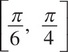is

3. Find the length of the arc of the curve defined by x =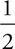t2 and y =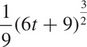, from t = 0 to t = 2.

4. The function f is given by f(x) = x4 + 4x3. On which of the following intervals is f decreasing?

5. Which of the following series converge(s)?

I.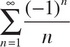II.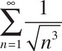III.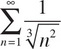6. Given the differential equation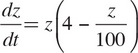, where z(0) = 50, what is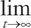z(t)?

7.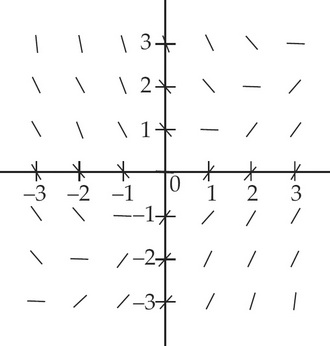The slope field shown above corresponds to which of the following differential equations?

8. The value of c that satisfies the Mean Value Theorem for derivatives on the interval [0, 5] for the function f(x) = x3 – 6x is

9.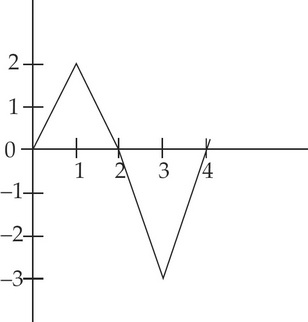The graph of f is shown in the figure above. If g(x) =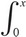f(t) dt, for what positive value of x does g(x) have a minimum?